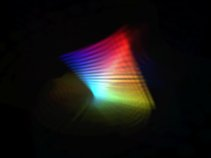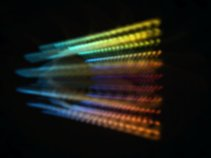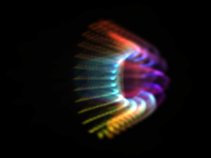Home RabidHaMsTeR.org ANIMATED POINT MORPH TUTORIAL for R2/ExtremeThis should give you a (very quick) start to making point morphs R2/Extreme 1.52 (and on)... I suggest you read the formula engine tutorial first. You could really do with a reasonable knowledge of maths for this - at least to know about 3D, and sin and cos... Intro In R2/Extreme, there are two kinds of of point morph - one is static, and the other is active. There is a utility with the Pro version to make static point morphs from images or from 3D models, but this covers making active point morphs, which can be done using just a text editor. R2/Extreme's point morphs are made using 360 points of light... for each one of these, a position in 3D (X,Y,Z) is needed (where roughly -1 < X,Y,Z < 1), as well as the colour (R,G,B,A) where (where 0 < R,G,B,A < 1). A stands for Alpha, which is how 'solid' the points are. 0=transparent, 1=solid, so you mostly keep A set to 1. Active Point Morphs are stored in the "WinAmp\Plugins\R2e\Data" directory in files with the extension '.aMorph'. On startup, R2e loads all of the .aMorph files, as well as the .Morph (static point morph) files. Starting... Obviously you're going to want to check how your point morph looks, and you don't want to have to wait until it comes around in the Point Morph scene, so its an idea to do the following: Start R2e, go to the Options screen, then Scene options, and set 'StartScene' to '1'. Close R2e, and in the 'R2e\Data' directory create another directory called 'Temp', and move all the .aMorph and .Morph files into this. Now once you've created a point morph file R2e will start and display your point morph straight away. Right... now all you have to do is make a point morph file. Open Notepad, and type in the following: [MAIN] count=1  FPoint=X=(2*D1)-1;Y=(2*D2)-1;Z=0;R=1;G=1;B=1;A=1; Row=20 In Notepad, click save as, select the R2e\Data directory, and type 'My Example.aMorph'. If you start R2Extreme now, a white grid of points should be displayed, that rotates around (if it doesn't rotate - upgrade R2Extreme to 1.52 or later). Remember to hit F3 straight away so that the scene doesn't change away from the point morph.Changing the point morph Now close R2e, and have a look at notepad again. "Row=20" is telling R2e that it is going to divide the 360 points up into a grid. The first side of the grid will have 20 points, the other will have whatever is left (360/20 = 18 points) so its an idea to make the number after row something that divides into 360 to produce a whole number (36,24,20,18,12,10 etc). If you don't want a grid of points, but just a strip (like for doing a ring) then set Row to 360. Now, R2e executes the formula after "FPoint=" once for each of the 360 points. If updates two variables, D1, and D2. D1 is a number to do with the first side of the grid of points, D2 is to do with the second. They both range from 0 to 1. You can see in the example formula "X=(2*D1)-1;Y=(2*D2)-1;Z=0;R=1;G=1;B=1;A=1;" whats happening. X is being set to "(2*D1)-1", which means the value that was from 0 to 1 is being turned into a value from -1 to 1. The same is happening with Y. Z is set to 0 so the grid is flat. The colours are simple set to all 1. Change "R=1;G=1;B=1;A=1;" so it reads "R=D1;G=D2;B=1-D1;A=1;", save, and restart R2e. You should now see that the grid is coloured differently. You can see whats happening here... at the first corner of the grid, D1=0 and D2=0, so the colours are R=0;G=0;B=1; = blue. At the opposite edge, D1=1 and D2=1, giving R=1;G=1;B=0; = yellow.Now to make our grid slightly more interesting. We're going to keep X the same, but change Y and Z so that we make a cylinder. If you've done A-level maths, you'll know that combining sin and cos in different directions produces a circle. In R2e, sin and cos work in radians, which is a value between 0 and 2*pi, so we must multiply our value D2 by 2*pi. So... replace "Y=(2*D2)-1;Z=0;" by "Y=sin(D2*2*pi);Z=cos(D2*2*pi);", start R2e and see what we get...Now for the 'active' bit. At the moment, our point morphs don't actually do anything. R2e provides some handy variables. One is "Time" - this is the time in seconds. As a demonstration of this, i'm going to turn the cylinder into kindof a cone (with a flat top) by multiplying Y and Z by (D1+1)/2 - so it will get bigger towards one end. I'm also changing X. It now doesn't go straight from one end to the other, its moved by sine, so there's kindof a curve to it, eg: "X=sin(D1*pi)/2". Notice i've only multiplied by pi, not 2*pi, so this is half a sine-wave. Now, to make it move. Suppose we want to make it wobble up and down? If we add "Time" to D1 ("X=sin((D1+time)*pi)/2") then the sine wave will move and the shape will 'wobble'. Our entire file now reads: [MAIN] count=1  FPoint=X=sin((D1+time)*pi)/2;Y=sin(D2*2*pi)*(D1+1)/2;Z=cos(D2*2*pi)*(D1+1)/2;R=D1;G=D2;B=1-D1;A=1; Row=20There isn't just "Time" to play with though. There is a constant called "Bass", which has an average value of about 1, and varies up and down with bass. There's also "BassTime", which is roughtly the time since the scene started, but when there's a bass beat it moves quicker and when there isn't it moves slower. Replacing "Time" with "BassTime" in the formula above would make the shape move quicker when there was a beat - often what you want. Random extra stuff I reckon thats actually about all you need to know. There is a tiny thing you ought to know, which is speed - the more complex the formulii the slower the framerate on R2e. I've done my best to make it quick, but there are one or two things you can do to help it. Formulii like "((D1*5)+1+2)*10" can be expanded to "(D1*50)+30" which does the same but is quicker. Note that brackets don't slow anything down, so feel free to put loads in to make stuff clearer and to make sure you know what the formula is going to do. Have a look at the other aMorph files in notepad and see if you can get some ideas - i find the best way to learn is to look at examples. I thought i'd just give a very small extra about using 'IF' to create different shapes...If you look above (yeah... its a bit blurry i know) you'll see there are two shapes, and different colours. This example isn't amazing, but it shows using the IF statement. Basically, IF looks at the first parameter - if its greater than 0 then it uses the second parameter, else the third. Be warned though - both bits are executed, and take time... In this case I use one long strip (Row=360) then divide it into two halves using "IF(D1-0.5, .. , .. )". [MAIN] count=1  FPoint=X=IF(D1-0.5,sin(D1*8*pi),0.5)*sin(D1*4*pi);Y=IF(D1-0.5,sin(D1*8*pi),0.5)*cos(D1*4*pi);Z=IF(D1-0.5,0.25,1)*sin(D1*16*pi);R=1;G=IF(D1-0.5,1,0);B=0;A=1; Row=360 Other stuff Well, hope that was sortof enough to get started... If you have any queries, please raise them on the R2 effects messageboard and i'll see if I can help you. Also, if you come up with a cool point morph please mail it to me and I might well be able to put it in the next release - you'll get full credit.site design by Gordon Williams